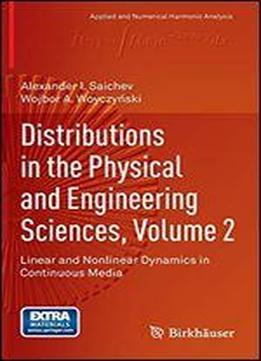Distributions In The Physical And Engineering Sciences, Volume 2: Linear And Nonlinear Dynamics In Continuous Media by Alexander I. Saichev / 2013 / English / PDF

Distributions in the Physical and Engineering Sciences is a comprehensive exposition on analytic methods for solving science and engineering problems. It is written from the unifying viewpoint of distribution theory and enriched with many modern topics which are important for practitioners and researchers. The goal of the books is to give the reader, specialist and non-specialist, useable and modern mathematical tools in their research and analysis. Volume 2: Linear and Nonlinear Dynamics of Continuous Media continues the multivolume project which endeavors to show how the theory of distributions, also called the theory of generalized functions, can be used by graduate students and researchers in applied mathematics, physical sciences, and engineering. It contains an analysis of the three basic types of linear partial differential equations elliptic, parabolic, and hyperbolic as well as chapters on first-order nonlinear partial differential equations and conservation laws, and generalized solutions of first-order nonlinear PDEs. Nonlinear wave, growing interface, and Burgers equations, KdV equations, and the equations of gas dynamics and porous media are also covered. The careful explanations, accessible writing style, many illustrations/examples and solutions also make it suitable for use as a self-study reference by anyone seeking greater understanding and proficiency in the problem solving methods presented. The book is ideal for a general scientific and engineering audience, yet it is mathematically precise. Features Application oriented exposition of distributional (Dirac delta) methods in the theory of partial differential equations. Abstract formalism is keep to a minimum. Careful and rich selection of examples and problems arising in real-life situations. Complete solutions to all exercises appear at the end of the book. Clear explanations, motivations, and illustration of all necessary mathematical concepts.

views: 161